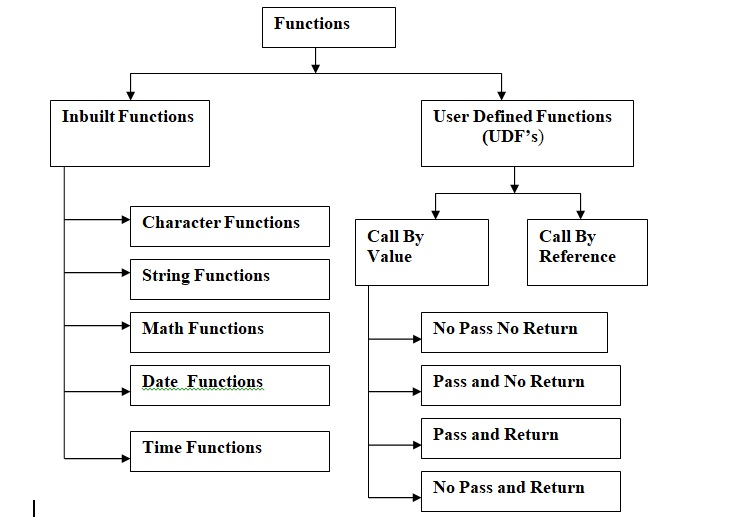C Language Tutorial Home

# C Language | Functions

What You Will Learn:

Def:  A function is a group/block of statements written to perform a specific task.

Each and every “C” program is made up of functions. There can be any number of functions in a single program. But there has to be at least one function in each and every program and if there is only one function in the program it will be the function named main() , because the execution of each and every program starts from the function named main().

• Function helps us to make the program modular in nature.
• Function helps us to divide a large program into number of smaller programs/modules.
• Different tasks of the large program can be performed by small programs/ modules / functions.
• It is easy to debug the program when functions are used.
• Reusability of code. Code once written can be reused again and again. Thus saving lot of time and space.
•  The function which calls another function is called the “calling function”.
• The function which is called is known as the “called function”.
• A function can call another function(any number of functions) from within itself.
• The process when a function calls itself is called recursion.

Classification of functionsInbuilt functions:

Inbuilt functions are the functions which are already present in the system. We don’t have to write programs related to them, we have to just use them.

Examples:

• Character functions
• String functions
• Math functions
• Date functions
• Time functions

User-defined functions :

UDF’s are the functions made by the programmer as per requirement. i.e. to perform a specific task.

• Call By value:
• In call by value we deal with actual values.
• Call By reference:
• In call by reference we deal with address i.e. pointers.

Call by value

Whenever we deal with functions following points related to the functions should be remembered.

Function declaration.
Function calling.
Function definition.

Function declaration

A function declaration is an indication to the system that the specified function is used later in the program. While giving function declaration we have to mention the following

1. Return type of the function i.e. the type of value of the function is returning back.
2.Name of the function.
3.The list of arguments being passed to the function i.e. list of data types and variable names(optional).
4. The function declaration is terminated by semicolon(;).

Syntax:
returntype function_name (list of arguments);

Example:

• No pass no return
• void abc();
• void xyz();
• Pass and no return
• void abc (int x, int y);
• void abc (int,int);
• void xyz(float,int);
• void xyz(int,float,char);
• pass and return
• int abc(int x,int y);
• float abc(int,int);
• no pass and return
• int abc();
• float abc();

Function calling

Function calling means invoking the function i.e. executing the function.
While calling the function we have to specify the following:
1.variable to receive the value, if function is returning some value (optional).
2.Name of the function being called.
3.List of values/variables/exp being passed to the function , if passed.
4.The function calling is terminated by the semicolon.

Syntax:
Variable=function_name(list of values/variables);

Example:

• No pass no return
• abc();
• xyz();
• pass and return
• a=abc(10,20);
• b=xyz(a,b);
• no pass and return
• a=abc();
• a=xyz();
• Pass and no return
• abc(10,20);
• abc(x,y);
• xyz(a*10,b-4);

Function definition

The function definition is a set of statements written to perform a specific task.
The function definition helps us to specify what and how the operation is performed.
The definition of the function is not terminated by semicolon.
The function definition can be given either above or below the calling function (main function).
If the definition of the function is given above the calling function [main ()] then giving function declaration becomes optional.

Syntax:
return_type function_name(list of arguments)
{
statements
return(value/variables/expression);
}

Example:

No pass No return

void abc()
{
statements;
statements;
}
void xyz()
{
Statement;
}

Pass and no return

void abc(int x,int y)
{
Statements;
Statements;
}
void xyz(float z,float b)
{
Statements;
Statements;
}

pass and return

int abc(int a,int b)
{
Statements;
Statements;
return(value/variable/exp);
}
float xyz(int a,int b)
{
Statements;
Statements;
return(value/variable/exp);
}

no pass and return

int abc()
{
Statements;
Statements;
return(value/variable/exp);
}
float xyz()
{
Statements;
Statements;
return(value/variable/exp);
}

User-Defined Functions

Call By value

NO PASS NO RETURN

In no pass no return, no value is passed from the calling function to the called function and no value is returned back from the called function to the calling function.

PASS AND NO RETURN

In Pass and no return, value(s) are passed from the calling function to the called function but no value is returned back from the called function to the calling function.

PASS AND RETURN

In Pass and Return, value(s) is/are passed from the calling function to the called function and a value is returned back from the called function to the calling function.

Note:A function can return a maximum of one value. There can be any number of return statements in a function but as soon as any one of return the statement gets executed the control goes back to the calling function.

No PASS AND RETURN

In No Pass and Return, no value is passed from the calling function to the called function but a value is returned back from the called function to the calling function.

### C Language Programming TutorialC Language Tutorial HomeIntroduction to C LanguageTokensIf Conditiongoto statement and LabelnameSwitch StatementsFor loop While LoopDo while loop break and continueFunctionsRecursionInbuild FunctionsStorage ClassesPreprocessorArraysPointers Structures and UnionsFile Handling Projects

Important PagesNumber Of Visitors